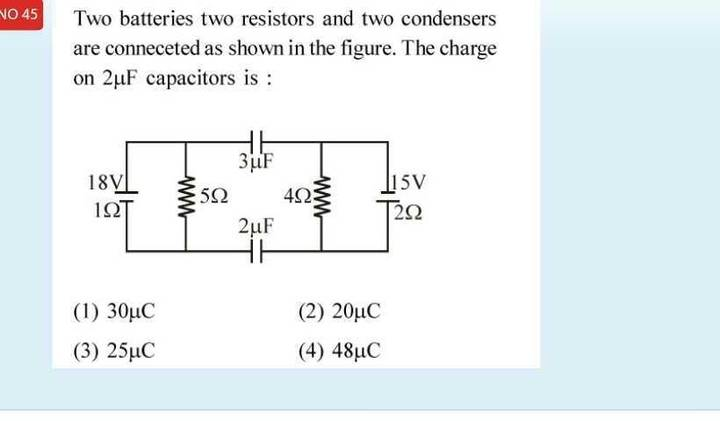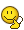Charge on a capacitor

Homework StatementThe Attempt at a Solution

In the steady state , there will be no current through the two branches containing the two capacitors . The capacitors can be replaced by open circuit .

I need to find potential difference across the two capacitors which will give the charges on the two capacitors.

There will be currents in the left and right loop .In steady state , the two loops will be independent of each other .

Since there is no common point in the two loops,which point should be assumed to have 0 reference potential ?

Attachments

haruspex
Homework Helper
Gold Member
which point should be assumed to have 0 reference potential ?
Potentials are always relative to some arbitrarily chosen zero. Pick any point you like, but be consistent.

Potentials are always relative to some arbitrarily chosen zero. Pick any point you like, but be consistent.

Agreed .

But in the steady state , the two loops will be independent . There will be no common point .

Homework Helper
Gold Member
@Jahnavi Suggestion: Since the capacitors are essentially an open circuit in the steady state, try computing the voltages from the top wire to the bottom wire that connects the two capacitors=on the left side and also on the right side. There is one more step you need to do once you compute that part, but try with that part first.

•Jahnavi
haruspex
Homework Helper
Gold Member
But in the steady state , the two loops will be independent
The only consequence of the currents is that they change the effective potentials produced by the batteries. As Charles posted, you can figure this out first.
Next, what is the relationship between the charge on the one capacitor and the charge on the other?

•Since the capacitors are essentially an open circuit in the steady state, try computing the voltages from the top wire to the bottom wire that connects the two capacitors=on the left side and also on the right side.

Call the top point of 5Ω A , bottom point B .

VA-VB = 15V

Call the top point of 4Ω C , bottom point D.

VD-VC = 10V

haruspex
Homework Helper
Gold Member
Call the top point of 5Ω A , bottom point B .

VA-VB = 15V

Call the top point of 4Ω C , bottom point D.

VD-VC = 10V
Good so far.

Homework Helper
Gold Member
Call the top point of 5Ω A , bottom point B .

VA-VB = 15V

Call the top point of 4Ω C , bottom point D.

VD-VC = 10V
Looks good. :) Now, for this next part you can ignore the ## 5 \Omega ## and ## 4 \Omega ## resistors. Take a look at the part you just computed. Can you make a circuit with it and the two capacitors? And as @haruspex asked in post 5, how is the charge on the two capacitors related?

•Jahnavi
Next, what is the relationship between the charge on the one capacitor and the charge on the other?

how is the charge on the two capacitors related?

I don't see why the charges on the two capacitors should be related to each other .

Homework Helper
Gold Member
I don't see why the charges on the two capacitors should be related to each other .
Any current that flows in these circuits must flow everywhere in a loop. Is there any way that the top capacitor can get a charge from current flowing into (and out of it) it that doesn't have the same current flowing into (and out of) the bottom capacitor?

•JahnaviOption a) i.e 30μC

•haruspex
Homework Helper
Gold MemberOption a) i.e 30μC
Looks right.

•Homework Helper
Gold MemberOption a) i.e 30μC
Hopefully you concluded that the charges on the capacitors are the same. The next step is to add the voltage drops around the outer loop and set the sum equal to zero. I presume that is what you did?

Can anyone help me find the right materials(online/offline) for studying more about solving these kinds of problems related to circuits

The next step is to add the voltage drops around the outer loop and set them equal to zero. I presume that is what you did?

No .

I added the two equations in post#6 , but this gives the same relation••